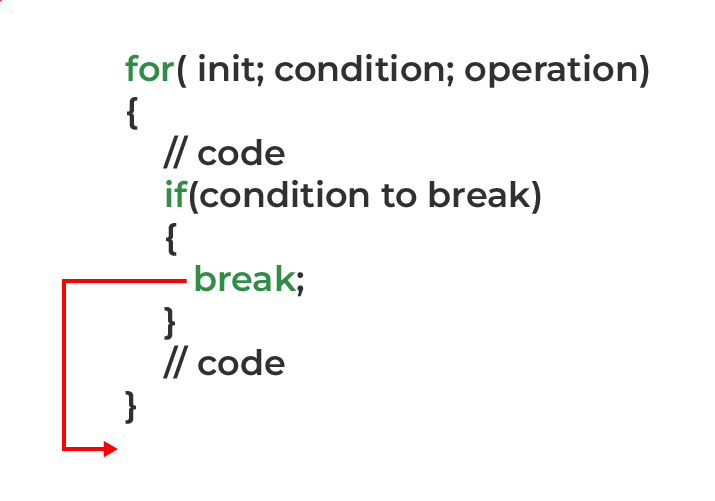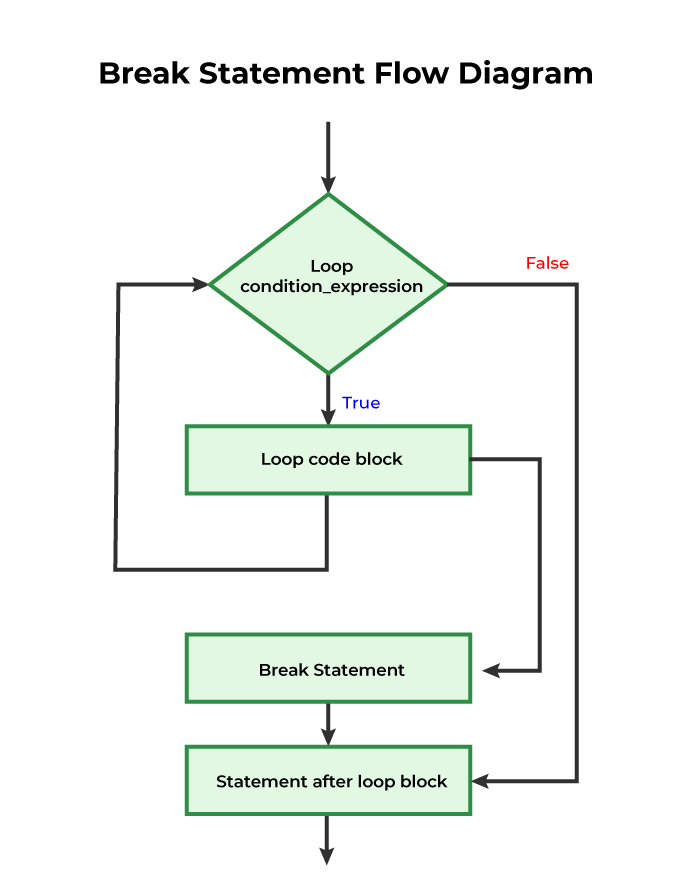# Break Statement in C

The break statement is one of the four jump statements in the C language. The purpose of the break statement in C is for unconditional exit from the loop

## What is break in C?

The break in C is a loop control statement that breaks out of the loop when encountered. It can be used inside loops or switch statements to bring the control out of the block. The break statement can only break out of a single loop at a time.

## Syntax of break in C

`break;`

We just put the break where ever we want to terminate the execution of the loop.

## Use of break in C

The break statement in C is used for breaking out of the loop. We can use it with any type of loop to bring the program control out of the loop. In C, we can use the break statement in the following ways:

• Simple Loops
• Nested Loops
• Infinite Loops
• Switch case

## Examples of break in C

### Example 1: C Program to use break Statement with Simple Loops

Break statements in C can be used with simple loops i.e, for loops, while loops, and do-while loops.

## C

 `// C Program to demonstrate break statement with for loop` `#include `   `int` `main()` `{`   `    ``// using break inside for loop to terminate after 2` `    ``// iteration` `    ``printf``(``"break in for loop\n"``);` `    ``for` `(``int` `i = 1; i < 5; i++) {` `        ``if` `(i == 3) {` `            ``break``;` `        ``}` `        ``else` `{` `            ``printf``(``"%d "``, i);` `        ``}` `    ``}`   `    ``// using break inside while loop to terminate after 2` `    ``// iteration` `    ``printf``(``"\nbreak in while loop\n"``);` `    ``int` `i = 1;` `    ``while` `(i < 20) {` `        ``if` `(i == 3)` `            ``break``;` `        ``else` `            ``printf``(``"%d "``, i);` `        ``i++;` `    ``}` `    ``return` `0;` `}`

Output

```break in for loop
1 2
break in while loop
1 2 ```

### Example 2: C Program to use break Statement with Nested Loops

Break statements can also be used when working with nested loops. The control will come out of only that loop in which the break statement is used.

## C

 `// C program to illustrate` `// using break statement` `// in Nested loops` `#include `   `int` `main()` `{` `    ``// nested for loops with break statement` `    ``// at inner loop` `    ``for` `(``int` `i = 1; i <= 6; ++i) {` `        ``for` `(``int` `j = 1; j <= i; ++j) {` `            ``if` `(i <= 4) {` `                ``printf``(``"%d "``, j);` `            ``}` `            ``else` `{` `                ``// if i > 4 then this innermost loop will` `                ``// break` `                ``break``;` `            ``}` `        ``}` `        ``printf``(``"\n"``);` `    ``}` `    ``return` `0;` `}`

Output

```1
1 2
1 2 3
1 2 3 4 ```

Note: Break statement only breaks out of one loop at a time. So if in nested loop, we have used break in inner loop, the control will come to outer loop instead of breaking out of all the loops at once. We will have to use multiple break statements if we want to break out of all the loops.

### Example 3: C Program to use break Statement with Infinite Loops

An Infinite loop can be terminated with a break statement as a part of the condition.

## C

 `// C Program to demonstrate infinite loop without using` `// break statement` `#include `   `int` `main()` `{`   `    ``int` `i = 0;`   `    ``// while loop which will always be true` `    ``while` `(1) {` `        ``printf``(``"%d "``, i);` `        ``i++;` `        ``if` `(i == 5) {` `            ``break``;` `        ``}` `    ``}` `    ``return` `0;` `}`

Output

`0 1 2 3 4 `

In the above program, the loop condition is always true, which will cause the loop to execute indefinitely. This is corrected by using the break statement. Iteration of the loop is restricted to 8 iterations using break.

## How break statement works?Working of break in a for loop

The working of the break statement in C is described below:

1. STEP 1: The loop execution starts after the test condition is evaluated.
2. STEP 2: If the break condition is present the condition will be evaluated.
3. STEP 3A: If the condition is true, the program control reaches the break statement and skips the further execution of the loop by jumping to the statements directly below the loop.
4. STEP 3B: If the condition is false, the normal flow of the program control continues.

## Flowchart of break in C## Break in C switch case

In general, the Switch case statement evaluates an expression, and depending on the value of the expression, it executes the statement associated with the value. Not only that, all the cases after the matching case after the matching case will also be executed. To prevent that, we can use the break statement in the switch case as shown:

### Syntax of break in switch case

```switch(expression)
{
case value1:
statement_1;
break;

case value2:
statement_2;
break;
.....
.....

case value_n:
statement_n;
break;

default:
default statement;
}```

## C

 `// C Program to demonstrate working of break with switch` `// case` `#include ` `#include `   `int` `main()` `{` `    ``char` `c;` `    ``float` `x, y;`   `    ``while` `(1) {` `        ``printf``(``"Enter an operator (+, -), if want to exit "` `               ``"press x: "``);` `        ``scanf``(``" %c"``, &c);` `        ``// to exit` `        ``if` `(c == ``'x'``)` `            ``exit``(0);`   `        ``printf``(``"Enter Two Values:\n "``);` `        ``scanf``(``"%f %f"``, &x, &y);`   `        ``switch` `(c) {` `        ``// For Addition` `        ``case` `'+'``:` `            ``printf``(``"%.1f + %.1f = %.1f\n"``, x, y, x + y);` `            ``break``;` `        ``// For Subtraction` `        ``case` `'-'``:` `            ``printf``(``"%.1f - %.1f = %.1f\n"``, x, y, x - y);` `            ``break``;` `        ``default``:` `            ``printf``(` `                ``"Error! please write a valid operator\n"``);` `        ``}` `    ``}` `}`

Output:

```Enter an operator (+, -), if want to exit press x: +
Enter Two Values:
10
20
10.0 + 20.0 = 30.0```

## Conclusion

In this article, we have discussed the break statement and how it can be used to alter the flow of the normal execution of the C program. The break statement is particularly useful when we want to terminate the loop earlier than the normal logic.

## FAQs on C break Statement

### 1. What is the use of a break statement in C?

The break statement in C is used for early termination and exit from the loop.

### 2. What is the difference between break and continue?

The difference between the break and continue in C is listed in the below table:

Whether you're preparing for your first job interview or aiming to upskill in this ever-evolving tech landscape, GeeksforGeeks Courses are your key to success. We provide top-quality content at affordable prices, all geared towards accelerating your growth in a time-bound manner. Join the millions we've already empowered, and we're here to do the same for you. Don't miss out - check it out now!

Previous
Next